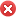#### 湖南工业大学湖南株洲市

• 官方网址：http://www.hut.edu.cn/
• 官方电话：0731-22183311,0731-22183355
• 电子邮箱：zsb@hut.edu.cn

\r

\r

\r

\r

\r

\r

\r

\r

\r

\r

\r

\r

\r

\r

\r

\r

\r

\r

\r

\r

\r

\r

\r

\r

\r

\r

\r

\r

\r

\r

\r

\r

\r

\r

\r

\r

\r

\r

\r

\r

\r

\r

\r

\r

\r

\r

\r

\r

\r

加载中~

## 男女比例52%48%98.6%

10.3%

7.4%

## 同类院校推荐## 报考指南